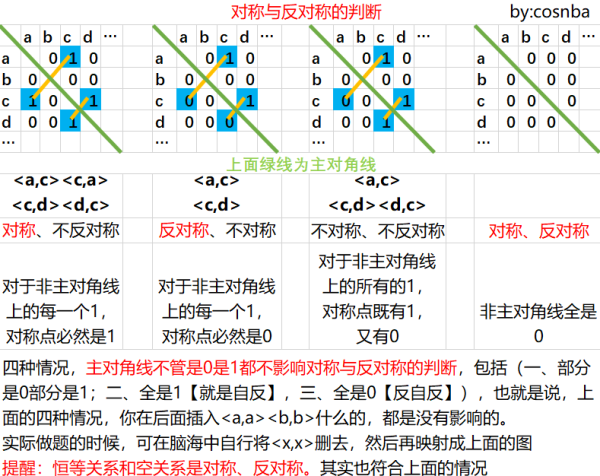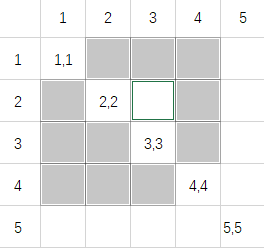Site Overlay

# 离散数学结构（抽象代数）笔记

## 基本概念

$\left| G \right|$ 记号 ：表示集合 $G$ 中元素的个数。

Dom：是定义域记号。

Ran：是值域记号。

1--->2
3----↑

a 的入度：就是有向图上指向 $a$ 的箭头数。

a 的出度：就是有向图上离开 $a$ 的箭头数。

a 到 b 的路径：是有向图上从 $a$ 到 $b$ 加上途径元素的一条有限序列 $a, x_1, x2, \cdots , x{n-1}, b$。算上 $a, b$ 一共是 $n+1$ 个元素。长度定为 $n$。（上图转自 笑一笑cat

p 决定的 A 上的等价关系

LUB： $a$ 是最小上界，就是没有比 $a$ 更小的上界 $a'$。

GLB： $a$ 是最大下界，就是没有比 $a$ 更大的下界 $a'$。

：如果一个偏序集，其任意两个元素构成的子集都有 LUB 和 GLB，那这个偏序集是一个格。$a \vee b$ 表示 $\operatorname{LUB}({a,b})$，$a \wedge b$ 表示 $\operatorname{GLB}({a,b})$。

【例子】 证明或者反证 $Z^+$ 上的 $a*b = \operatorname{GCD}(a,b)$ 具有幂等性质。

【分析与解答】 即证 $a = \operatorname{GCD}(a, a)$ 对于所有 $a \in Z^+$ 成立。由于 $a / a = 1$ 余数是 $0$，所以 $\operatorname{GCD}(a,a) = a$，所以是幂等的。

### 为什么陪集是等价类？

$(ga)^{-1}gb = a^{-1} g^{-1} g b = a^{-1} (g^{-1} g) b = a^{-1}b \in H$。所以 陪集中任意两个元素之间都存在R这个等价关系，所以 $g$ 在 $H$ 的陪集就是等价类 $[g]$。所有陪集($g_1H, g_2H, \cdots, g_nH$)就是等价类的集合——构成了 $G$ 的划分（等价类不可能相交，如果重叠就会归为同一个等价类。也不会遗漏，因为对于 $G$ 中每个元素，我们都得到了它的等价类）。

### 如何证明同态？

【例子】 证明:函数$f(x)=|x|$是从非零实数群$G$到正实数群$G'$的一个同态，其中群$G$和$G'$中的运算是乘法。

$f(ab) = |ab| = |a||b| = f(a)f(b)$。

【例子】 33．设G是一个群。证明:由$f(a)=a^2$定义的函数 $f:G→G$ 是一个同态当且仅当 $G$ 是阿贝尔群。

【分析与解答】

$f(ab) = (ab)^2 = b^2 a^2 = bbaa$

$f(a) f(b) = a^2 b^2 = aabb$

### 如何证明同构

【例子】 35．设G是一个群，a是G中的一个固定元素。证明:对于 $x \in G$ ，由 $f(x)=axa^{-1}$ 定义的函数 $f_a:G→G$ 是一个同构。

【分析与解答】 $f(x)f(y) = axa^{-1} aya^{-1} = axy^{-1}a = f(xy)$。所以运算法则是一致的。然后证明双射吧。

### 如何证明正规子群

1. $H \triangleleft G$
2. 左右陪集相等：$gH = Hg, \forall g \in G$
3. $\forall g_1, g_2 \in G, g_1H \cdot g_2H = g_1g_2H$

### 其它

【例子】 对于 $n$ 元素集合，可构成多少个自指关系？

【分析与解答】 首先我们有前提：$n$ 个元素构成的任何集合都是 $n\times n$ 的子集。【例子】 Let A be a set with n elements. How many commutative binary operations can be defined on A?

【分析与解答】

$n^{n^2}$